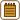## Entropy change of helium

### Task number: 1293

Helium stored in a container under pressure 10 MPa starts to leak slowly through a broken valve until its pressure drops to the atmospheric pressure 101 325 Pa. The whole process proceeds isothermally at room temperature 20 °C. Find the change in entropy for this ideal gas of weight 1.0 kg.

• #### Notation

 p1 = 10 MPa = 107 Pa initial helium pressure in the container p2 = 101325 Pa pressure after the helium leakage m = 1 kg helium weight t = 20 °C => T = 293.15 K process temperature ΔS = ? change in helium entropy

From tables:

 Mm = 4 g mol−1 = 4·10−3 kg mol−1 helium molar weight R = 8.31 JK−1mol−1 molar gas constant
• #### Hint 1 – What kind of process occurs?

The container does not change its volume, therefore we could consider a gas process with constant volume but variable weight. This way, only the entropy change inside the container would be found but not the whole change in the entropy.

Instead, the whole amount of the gas will be considered, i.e. with constant weight. You can imagine that the leaking gas expands into the “close vicinity” of the container.

• #### Hint 2 – Change in entropy ΔS

The elemental entropy is defined by the following relation:

$\mathrm{d}S=\frac{\mathrm{d}Q}{T},$

where dQ is the elemental exchanged heat and T denotes the thermodynamic temperature.

Because it is an isothermal process, i.e. T = konst., the previous equation could be “integrated” easily. To evaluate the change in entropy ΔS, we obtain

$\Delta S=\frac{Q}{T},$

where Q stands for the overall exchanged heat.

• #### Hint 3 – Expression of heat

One has to realize, that gas internal energy remains constant at constant temperature, therefore the heat accepted during the expansion equals the work done by the gas.

• #### Hint 4 – Calculation of work

Think over how to calculate the work done by the gas if its pressure changes during the process (it is a function of volume).
• #### Hint 5 – Pressure p(V) expression

To express the pressure p as a function of the volume V, the so‑called Boyle‑Mariotte law could be applied.

• #### Hint 6 – Volume expression

To find the unknown initial V1 and final V2 volumes, the equation of state will be applied:

$pV=\frac{m}{M_m}RT,$

where m is the weight, Mm the molar weight, T the gas temperature and R the molar gas constant.

• #### Analysis

Since we consider an isothermal process, the change in entropy will be calculated as a fraction of the overal heat and the thermodynamic temperature at which the process takes place.

During the isothermal process, the internal energy of gases remains constant, thus the heat accepted during an expansion equals the work done by the gas. To calculate this work, the integral calculus has to be applied, because the pressure is a function of the volume. The pressure as a function of the volume could be expressed from the Boyle-Mariotte law.

To find the unknown initial and the final volume, the equation of state for an ideal gas will be employed.

• #### Solution

Since we have an isothermal process, we can find the change in entropy ΔS by applying the following relation:

$\Delta S=\frac{Q}{T},$

where Q is the overall heat and T denotes the thermodynamic temperature, at which the process takes place.

During an isothermal process, the gas internal energy remains constant. According to the 1st thermodynamic law, the accepted heat Q will equal the work W done by the gas which agrees with the 1st thermodynamic law.

This work could be found from the following relation:

$W=\int\limits_{V_1}^{V_2}p\,\text{d}V,$

where V1 and V2 are the initial and the final gas volume and p is the system pressure which changes during the expansion (it is a function of the volume V).

To express the pressure p as a function of the volume V, the Boyle-Mariotte law will be applied:

$p_1V_1=pV.$

The pressure p is found:

$p = \frac{p_1V_1}{V}.$

Actual integration:

$W = \int\limits_{V_1}^{V_2}p\, \text{d}V = \int\limits_{V_1}^{V_2}\frac{p_1V_1}{V}\, \text{d}V =$

Constants are factored out in front of the integral

$=p_1V_1 \int\limits_{V_1}^{V_2}\frac{1}{V}\, \text{d}V =$

It is integrated then and the limits are recalled.

$=p_1V_1\,[\ln V]_{V_1}^{V_2} = p_1V_1\,\ln\,\frac{V_2}{V_1}.$

We will express the unknown initial V1 and the final V2 volume from the equation of state:

$p_1V_1=\frac{m}{M_m}RT \qquad \Rightarrow \qquad V_1=\frac{mRT}{p_1M_m},$ $p_2V_2=\frac{m}{M_m}RT \qquad \Rightarrow \qquad V_2=\frac{mRT}{p_2M_m}.$

After recall:

$W=p_1\frac{mRT}{p_1M_m}\,\ln{\frac{\frac{mRT}{p_2M_m}}{\frac{mRT}{p_1M_m}}}=\frac{mRT}{M_m}\,\ln{\frac{p_1}{p_2}}.$

The searched change in entropy is then:

$\Delta S=\frac{Q}{T}=\frac{W}{T} = \frac{\frac{mRT}{M_m}\,\ln{\frac{p_1}{p_2}}}{T}=\frac{mR}{M_m}\,\ln{\frac{p_1}{p_2}}.$
• #### Numerical Evaluation

$\Delta S= \frac{m}{M_m}R\,\ln\,{\frac{p_1}{p_2}}$ $\Delta S= \frac{1{,}0}{4.0\cdot{ 10^{-3}}}\cdot{ 8.31}\cdot \ln{\left(\frac{10^7}{101\,325}\right)}\,\mathrm{JK^{-1}}\dot{=}9\,500\,\mathrm{JK^{-1}}$
• #### Answer

The gas entropy increases to approximately 9500 JK−1.